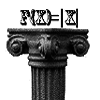#### You may also like### Equation Matcher

Can you match these equations to these graphs?### Maths Shop Window

Make a functional window display which will both satisfy the manager and make sense to the shoppers

# Real-life Equations

### Why do this problem?

This problem encourages students to get into the real meaning of equations and graphical representation without getting bogged down in algebraic calculations or falling back into blind computation. It will help to reinforce the differences between different 'types' of equation.

### Possible approach

Note the difference between showing that an equation is a possibility and showing that it is not a possibility. In the first case, students need only give a single example of a curve with certain paramaters which passes through a point of the required type. To show that an equation CANNOT pass through a point of a certain type requires more careful explanation. Hopefully students will work this out for themselves, but prompt them if necessary.

### Key questions

• How can you tell if a certain point will match a certain equation type?
• How can you tell if a certain point will not match a certain equation type?

### Possible extension

You might naturally try Equation matching next.

### Possible support

Give concrete examples by labelling the points $(1, -1), (-1, 1), (-1, -1), (1, -1)$

Alternatively, try the easier non-algebraic question Bio-graphs